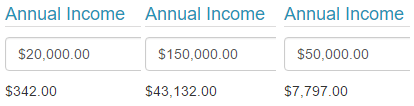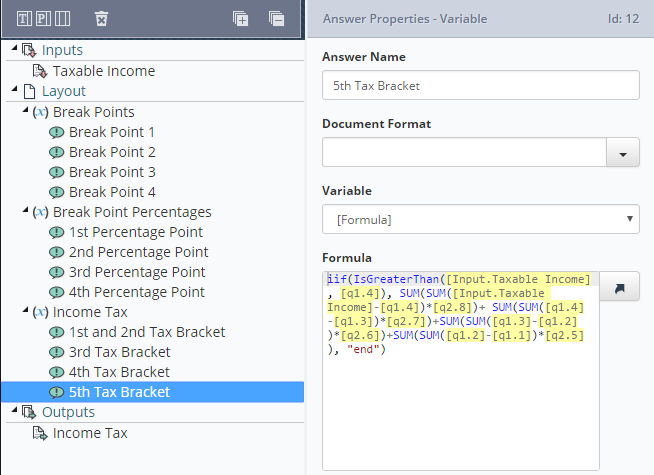# Overview

In this tutorial, we are going to be building a fragment which will calculate income tax. This tutorial guide will be using the income data from the 2016-2017 financial year.

### 🚧Warning

This exercise assumes you have completed the advanced training.

In this guide we will be learning how to:

• Create a nested iif statement
• Using iif statements to reference other iif statements

# Creating the Data Source

• Create a new project
• Call the Project: Fp Australian Income Tax Calculator
• Set the ProjectType to Fragment Portion
• Drop a Variable into the Layout and call it Break Points. Add up to 4 answers and call them,
``````Break Point 1, Break Point 2, Break Point 3 and Break Point 4.
``````
• Set the following formulas in order of the Break Points 1-4:
``````Concat(“18200”), Concat(“37000”), Concat(“87000”), Concat(“180000”)
``````
• Add another Variable and call it Break Point Percentages
• Add 4 answers and call them, 1st Percentage Point, 2nd Percentage Point, 3rd Percentage Point and 4th Percentage Point.

Set the following formulas to:

``````Concat(“0.19”), Concat(“0.325”), Concat(“0.37”), Concat(“0.45”)
``````
• Add another Variable and call it Income Tax
• Add 4 answers and call them, “1st and 2nd Tax Bracket”, 3rd Tax Bracket, 4th Tax Bracket, and 5th Tax Bracket.
• Before we add any formulas to the variables, add an Input and call it Taxable Income.

### 📘Note:

The way Australian tax brackets work is that you have to pay a certain amount of tax for each bracket.

Let’s say someone earns \$90,000.

The first \$18,200 they earn will be free from income tax.

The next bracket is between \$18,201 and \$37,000 and has a tax percentage of 19c per the dollar. In other words, the next \$18,799 is taxed at 19%.

The next bracket is between \$37,001 and \$87,000. So the next \$50,000 is taxed at 32.5%.

The next bracket is between \$87,001 and \$180,000. In our example, because the man only earns \$90,000, only the next \$3,000 will be taxed at the new bracket.

The last tax bracket does not apply to the person because they earn under \$180,001.

In our formulas, we will have to make a tax deduction for each bracket and work out when to swap to the next bracket.

• Inside Income Tax – 1st and 2nd Tax Brackets, add the following formula:
``````iif(IsLessThanOrEqualTo([Input.Taxable Income], [q1.1]), "0", "This is above the first bracket")
``````

[q1.1] references Break Point 1 in this case.

What this formula does, is ask if the input is less than or equal to Break Point 1. If this is true, then the answer is equal to 0, otherwise, the answer is equal to the string “This is above the first bracket”.

In this case, this is not what we want, as we want to continue the calculations. There are two ways of doing this. We can either create a nested iif statement or reference another question that asks a follow-up question.

For 1st and 2nd Tax Bracket, we are going to continue the formula with a nested iif statement.

Edit the formula to this:

``````iif(IsLessThanOrEqualTo([Input.Taxable Income], [q1.1]), "0", iif(IsLessThanOrEqualTo([Input.Taxable Income], [q1.2]), SUM(SUM([Input.Taxable Income]-[q1.1])*[q2.5]), [q3.10]))
``````

What this formula does ask is the income is less or equal to [q1.1] Break Point 1, if it is it will equal “0”, if not it will ask if it is less than [q1.2] Break Point 2. If this is true it will Subtract [q1.1] Break Point 1 from the [Input.Taxable Income] Input and then multiply it by [q2.5] 1st Percentage Point.
If this is false it will reference the next question which is [q3.10] 3rd Tax Bracket.

### 📘Note:

It is possible to have as many nested iif statements as you can fit inside of a single formula but a lot of the time this can end up with a crowded and messy formula. This can lead to mistakes being more easily made, as well as identifying a point of failure being more difficult as well. This is why we are referencing another variable answer, too make sure the formulas are clean and easy to understand.

• In the 3rd Tax Bracket, enter the following formula.
``````iif(IsLessThanOrEqualTo([Input.Taxable Income], [q1.3]), SUM(SUM([Input.Taxable Income]-[q1.2])*[q2.6])+SUM(SUM([q1.2]-[q1.1])*[q2.5]), [q3.11])
``````

In this formula, we ask if the taxable income is less than or equal to [q1.3] Break Point 3. If it is true we tax the [Input.Taxable Income] Input subtracting [q1.2] Break Point 2 and then multiply it by [q2.6] 2nd Percentage Point and then add the Sum of [q1.2] Break Point 2 minus [q1.1] Break Point 1 multiplied by [q2.5] 1st Percentage Point. If false we reference [q3.11] 4th Tax Bracket.

• In the 4th Tax Bracket, enter the following formula:
``````iif(IsLessThanOrEqualTo([Input.Taxable Income], [q1.4]), SUM(SUM([Input.Taxable Income]-[q1.3])*[q2.7])+ SUM(SUM([q1.3]-[q1.2])*[q2.6])+SUM(SUM([q1.2]-[q1.1])*[q2.5]), [q3.12])
``````

By now you will see a trend of the formulas adding the full taxed amounts from the lower brackets onto the amount taxed at the highest bracket.

• In 5th Tax Bracket, enter the following formula:
``````iif(IsGreaterThan([Input.Taxable Income], [q1.4]), SUM(SUM([Input.Taxable Income]-[q1.4])*[q2.8])+ SUM(SUM([q1.4]-[q1.3])*[q2.7])+SUM(SUM([q1.3]-[q1.2])*[q2.6])+SUM(SUM([q1.2]-[q1.1])*[q2.5]), "Error")
``````

In this formula, we are asking if the input value is greater than [q1.4] Break Point 4. This is because it is the highest bracket. Because it is the highest break it also doesn’t have another question to reference at the end.

It should also be impossible for the formula to be false, but in case this does occur we just leave a simple “Error” if something has gone wrong.

• Add an output to the project.
• Call the output, Income Tax and insert the following properties:

Question: Income Tax
Answer: 1st and 2nd Tax Bracket

• Save the project.

We have just created a fragment that will calculate the income tax a person has to pay, however, we need to test it.

• Create a new Project and call it Income Tax Calculator.
• Insert a Text field onto the question set and call it Annual Income.
• Set its Data type to Currency
• Add the fragment we just created onto the question set.

Before we link the Input to the Text field we will want to add a variable above the fragment.

• Call both the question and answer Change to General Number.
• Type in the formula format([q1], “G”)

We do this to make sure the input can be used for math.

• In fragment inputs, set it so that Taxable Income references format to General Number.
• Add another variable below the fragment and call the question and answer, Change to Currency.
• Type in the formula Format([Fragment.Fp_Australian Income Tax Calculator.Income Tax], "C")
• Add a Label and call it Total Taxed amount
• Reference Format to Currency – Format to Currency.
• In Conditions, make it so that it is only active if Annual Income does not equal nothing.
• Save and publish the project.

You should now have a working income tax calculator.

Example of outputs you should receive.Example of what the fragment should look like.